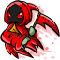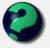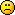# Math Is Fun Forum

Discussion about math, puzzles, games and fun.   Useful symbols: ÷ × ½ √ ∞ ≠ ≤ ≥ ≈ ⇒ ± ∈ Δ θ ∴ ∑ ∫  π  -¹ ² ³ °

You are not logged in.

## #1 2005-05-21 06:21:20

i am evil, always will be
Member
Registered: 2005-05-21
Posts: 4

### 0.9999....(recurring) = 1?

i noticed this some time ago and wondered how long it had been on mathsisfun.com.
plz can you tell me because i happen to know some1 who also discovered this and would like to know who found it first. also do any of you agree or disagree with this theory? i would like to know your thoughts on it, thanks.

Offline

## #2 2005-05-21 11:04:00

MathsIsFunRegistered: 2005-01-21
Posts: 7,710

### Re: 0.9999....(recurring) = 1?

It has been on the site for some years now ....

I think some research would find this concept has been around for 100s of years.

What is so cool about it is the disagreement it causes! For example here

"The physicists defer only to mathematicians, and the mathematicians defer only to God ..."  - Leon M. Lederman

Offline

## #3 2005-05-21 21:02:58

Zach
MemberRegistered: 2005-03-23
Posts: 2,075

### Re: 0.9999....(recurring) = 1?

I've done that with 0.27 recurring. It's quite a logical thought.Boy let me tell you what:
I bet you didn't know it, but I'm a fiddle player too.
And if you'd care to take a dare, I'll make a bet with you.

Offline

## #4 2005-07-01 18:04:57

ganeshRegistered: 2005-06-28
Posts: 38,066

### Re: 0.9999....(recurring) = 1?

0.1111111111111.....(recurring) can be written as a fraction
as 1/9.
When the recurring decimal is multiplied by 9,
we get 0.99999999999999999999........ (recurring).
But when we perform the same operation
on the number expressed as a fraction,
we have to multiply 9 and 1/9.
We get 1.
Therefore,
0.9999999999999999.........(recurring)
is equal to 1.

It appears to me that if one wants to make progress in mathematics, one should study the masters and not the pupils. - Niels Henrik Abel.

Nothing is better than reading and gaining more and more knowledge - Stephen William Hawking.

Offline

## #5 2005-07-01 19:25:54

MathsIsFunRegistered: 2005-01-21
Posts: 7,710

### Re: 0.9999....(recurring) = 1?

Neat and concise ... thanks ganesh.

"The physicists defer only to mathematicians, and the mathematicians defer only to God ..."  - Leon M. Lederman

Offline

## #6 2005-08-09 10:28:55

MathsIsFunRegistered: 2005-01-21
Posts: 7,710

### Re: 0.9999....(recurring) = 1?

So, the limit of 0.999... =1

But 0.999... = 1 - infinitesimal

Correct, O Master of the Infinite?

"The physicists defer only to mathematicians, and the mathematicians defer only to God ..."  - Leon M. Lederman

Offline

## #7 2005-08-09 16:20:35

ganeshRegistered: 2005-06-28
Posts: 38,066

### Re: 0.9999....(recurring) = 1?

I thought of it in a different way. I used the summation of a Geometric Progression and this is the result I got:-
0.9999999999999999999999999999.....................
= 0.9(1 + 1/10 + 1/100 + 1/1000 + 1/10,000 + 1/100,000 + 1/1,000,000.......)
The ∑ of the series within the brackets would be 1/(1-1/10) = 1/(9/10)
= 10/9
10/9 x (0.9) = 9/9 = 1
I know this is an approximation for an infinite series.
But 0.999999999999999999999999999999999.................... is a devilish number. You never encounter this number in any mathematical problem. I have never come across recurring 9s after the decimal in any division. It can be proved that there can be no recurring 9s in any division.
Let me prove this by a counter-solution.
Let there be a recurring decimal 0.2999999999999999999.......
When we try to convert this into a fraction, this is what happens.
x = 0.2999999999999999999............
10x = 2.999999999999999999999......
100x = 29.9999999999999999999999......
90x = 27
x = 27/90 = 3/10, which is 0.3 !
Therefore, I think 0.9999999999999..........exists only theoretically. Only in the imagination.

It appears to me that if one wants to make progress in mathematics, one should study the masters and not the pupils. - Niels Henrik Abel.

Nothing is better than reading and gaining more and more knowledge - Stephen William Hawking.

Offline

## #8 2005-08-09 21:08:42

wcy
Member
Registered: 2005-08-04
Posts: 117

### Re: 0.9999....(recurring) = 1?

Another simple way of doing it is to let x=0.9999999

then, 10x=9.9999999999

Equation (2)-(1),
9x=9
x=1

I am not sure if this is mathematically correct

Offline

## #9 2005-08-10 22:39:33

i am evil, always will be
Member
Registered: 2005-05-21
Posts: 4

### Re: 0.9999....(recurring) = 1?

woah woah woah woah woah!!! slow down people! i'm thirteen! how the heck am i meant to have a clue bout what ur on about? can anyone explain SIMPLY? by the way my maths teacher says that if i like something on the scale of one to ten i can have 10.1!?!?!?! is that right? how? help!!!!!!!!!!

Offline

## #10 2005-08-10 22:41:22

i am evil, always will be
Member
Registered: 2005-05-21
Posts: 4

### Re: 0.9999....(recurring) = 1?

"The physicists defer only to mathematicians, and the mathematicians defer only to God ..."  - Leon M. Lederman

I think you mean to say differ not defer

Offline

## #11 2005-08-10 23:40:22

MathsIsFunRegistered: 2005-01-21
Posts: 7,710

### Re: 0.9999....(recurring) = 1?

Well, evil, your question simply got a lot of us interested! Once started, a topic takes on a life of its own ...

The answer, in a nutshell, is that 0.9999....(recurring) does not equal 1, because it misses out by an "infinitesimal" amount.
______

On a scale of one to ten, the lowest value is 1, the highest is 10, I would have thought. Why not ask your maths teacher to come on to the forum one day and they can explain how you get 10.1!
______

(de·fer  v.  1. To submit to the opinion, wishes, or decision of another)

"The physicists defer only to mathematicians, and the mathematicians defer only to God ..."  - Leon M. Lederman

Offline

## #12 2005-08-11 16:38:43

ganeshRegistered: 2005-06-28
Posts: 38,066

### Re: 0.9999....(recurring) = 1?

kylekatarn wrote:

I don't know if the way I represent the infinitesimal quantity ( 0.(0)1 ) is mathematically correct. But I found it very usefull while researching on this.

0.(0)1 is 1/(10^ ∞)
When I had to deal with numbers like 9.9999999999999999999....8,
I put a horizontal arrow pointing towards the right above the 9 after the decimal.Then I put the number 8. This meant that 9 keeps on recurring but the last digit is 8. But your method seems better, more convenient. Whether it would be accepted by Mathematicians worldover is another thing!

But the point I wanted to make is 0.999999999999...................... is a devilish number and would never be found in any mathematical problem or solution. It cannot be represented in a graph, It cannot be said to be irrational, yet it cannot be expressed as a rational number. It is not a transcendental number. As a rule, it should be possible to represent all decimals as a rational number. But this number defies the rule, although it is a recurring decimal. Sin 89.99999999999999999999999999999........ degrees can be said to be equal to this number.

I give up..........

It appears to me that if one wants to make progress in mathematics, one should study the masters and not the pupils. - Niels Henrik Abel.

Nothing is better than reading and gaining more and more knowledge - Stephen William Hawking.

Offline

## #13 2005-08-11 17:55:04

MathsIsFunRegistered: 2005-01-21
Posts: 7,710

### Re: 0.9999....(recurring) = 1?

1/3 = 0.333...
3 x (1/3) = 1

I dare not go any further ...

"The physicists defer only to mathematicians, and the mathematicians defer only to God ..."  - Leon M. Lederman

Offline

## #14 2005-08-11 20:47:58

mathsyperson
ModeratorRegistered: 2005-06-22
Posts: 4,900

### Re: 0.9999....(recurring) = 1?

In kylekatarn's proof that 0.999... ≠ 1, the one in the second picture of his big post, he says near the start that x=0.999... and that x=1-. Doesn't that mean that he's using the as-yet-unproven-fact that 0.999... ≠ 1 in order to prove that 0.999... ≠ 1? If so, surely that's not allowed.

Why did the vector cross the road?
It wanted to be normal.

Offline

## #15 2005-08-11 20:58:25

MathsIsFunRegistered: 2005-01-21
Posts: 7,710

### Re: 0.9999....(recurring) = 1?

If kyle is right, I have a  page to redo. Have a read of this. (I think this is the original page that "evil" found.)

I had thought it was right up until now."The physicists defer only to mathematicians, and the mathematicians defer only to God ..."  - Leon M. Lederman

Offline

## #16 2005-08-11 20:59:54

wcy
Member
Registered: 2005-08-04
Posts: 117

### Re: 0.9999....(recurring) = 1?

no matter what, 0.99999999999... is really close to 1

here is another "proof" thought of by me
Let
a=1
b=0.0000000000000000...1
a-b=0.99999999....
a²-b²=1²-0.000000....1=0.99999999...
(a+b)(a-b)=a²-b²
a+b = (a²-b²)/(a-b)=0.9999.../0.9999...=1
a=1
therefore b=0 !!!!
therefore a-b=0.999999...=1-0=1  !!!!!

Offline

## #17 2005-08-11 21:05:30

mathsyperson
ModeratorRegistered: 2005-06-22
Posts: 4,900

### Re: 0.9999....(recurring) = 1?

Looks OK to me. You're still assuming that 0.999... ≠ 1, but it's OK this time because you're doing a proof by contradiction.
Also, you might want to turn smilies off.

Why did the vector cross the road?
It wanted to be normal.

Offline

## #18 2005-08-11 21:33:59

MathsIsFunRegistered: 2005-01-21
Posts: 7,710

### Re: 0.9999....(recurring) = 1?

[I fixed ganesh's accidental smiley by putting in spaces]

So, I am still not sure how to edit that page ...

"The physicists defer only to mathematicians, and the mathematicians defer only to God ..."  - Leon M. Lederman

Offline

## #19 2005-08-11 22:42:23

ganeshRegistered: 2005-06-28
Posts: 38,066

### Re: 0.9999....(recurring) = 1?

wcy wrote:

a = 1
b=0.0000000000000000...1
a-b=0.99999999....
a²-b²=1²-0.000000....1=0.99999999...
(a+b)(a-b)=a²-b²
a+b = (a²-b²)/(a-b)=0.9999.../0.9999...=1
a=1
therefore b=0 !!!!
therefore a-b=0.999999...=1-0=1  !!!!!

If we are talking of infinite number of zeros after the decimal, before the 1, in b,
the proof is correct!
Because, a²-b² would be equal to a-b.
This is because we have defined 0.0000000000.......1 as 1 ater an infinite number of zeros after the decimal followed by 1,
as kylekatarn denotes 0.(0)1.

However, if the number of zeros is finite, (Don't worry, it is not)
a²-b² would contain more 9s than a-b.

It appears to me that if one wants to make progress in mathematics, one should study the masters and not the pupils. - Niels Henrik Abel.

Nothing is better than reading and gaining more and more knowledge - Stephen William Hawking.

Offline

## #20 2005-08-12 02:23:36

mathsyperson
ModeratorRegistered: 2005-06-22
Posts: 4,900

### Re: 0.9999....(recurring) = 1?

The basic proof of x=0.999..., 10x=9.999..., 9x=9, x=1 relies on the amount after the decimal point for x and 10x being the same.
If 10x= 9.(infinity of 9's), then x would be 0.(infinity of 9's +1), but infinity is the largest number possible, so infinity+1=infinity.

Why did the vector cross the road?
It wanted to be normal.

Offline

## #21 2005-08-12 10:59:50

MathsIsFunRegistered: 2005-01-21
Posts: 7,710

### Re: 0.9999....(recurring) = 1?

I tend to agree with you, kyle, the proof I have on the website has that niggly little thing that assumes that:

``````If x=0.999... , and 10x = 9.999...

Then the fractional part of 9.999... is still the SAME 0.999... as x=0.999...``````

So perhaps that is a flawed proof.

I want to replace it with something new, but it needs to appeal to a wide audience, so possibly we could condense the interesting arguments here into something really good.

"The physicists defer only to mathematicians, and the mathematicians defer only to God ..."  - Leon M. Lederman

Offline

## #22 2005-08-12 23:03:18

wcy
Member
Registered: 2005-08-04
Posts: 117

### Re: 0.9999....(recurring) = 1?

actually this problem is only relevant to mathematics.

In physics and chemistry, 1+a=1 if a is small.

Offline

## #23 2005-08-13 19:08:54

wcy
Member
Registered: 2005-08-04
Posts: 117

### Re: 0.9999....(recurring) = 1?

This proof will probably prove once and for all that 0.999..=1

0.9999...=0.9+0.09+0.009+0.0009+...
This is clearly a Geometric Progression, so we can use the formula Sum to infinity=a/(1-r), where a is the first term and r is the common ratio (0.1)

Hence, the sum to infinity=0.9/(1-0.1)=0.9/0.9=1 !!!

Proof of GP formula:
(1-r)(a+ar+ar^2+ar^3+...)=a
Therefore, a+ar+ar^2+ar^3+...=a/(1-r)

Offline

## #24 2005-08-14 09:38:10

MathsIsFunRegistered: 2005-01-21
Posts: 7,710

### Re: 0.9999....(recurring) = 1?

It comes down to "what does 0.999... mean"

First of all, we have been writing 0.999... to mean "zero-point-nine-recurring". (Normally this would be written as 0.9 with a little dot above the 9, but it is hard to write that dot)

So, does 0.999... = the limit of the infinite series 9/10 + 9/100 + 9/1,000 + ... ?

Or, does it just mean the series itself (not the limit) ?

"The physicists defer only to mathematicians, and the mathematicians defer only to God ..."  - Leon M. Lederman

Offline

## #25 2006-09-22 09:19:04

junior
Member
Registered: 2006-09-22
Posts: 2

### Re: 0.9999....(recurring) = 1?

Basic proof for those of you who are confused:

x = 0.999...
10x = 9.999...
9x = 10x - x = 9
9x ÷ 9 = x
9 ÷ 9 = 1

∴ x = 1
∴ 0.999... = 1

Offline